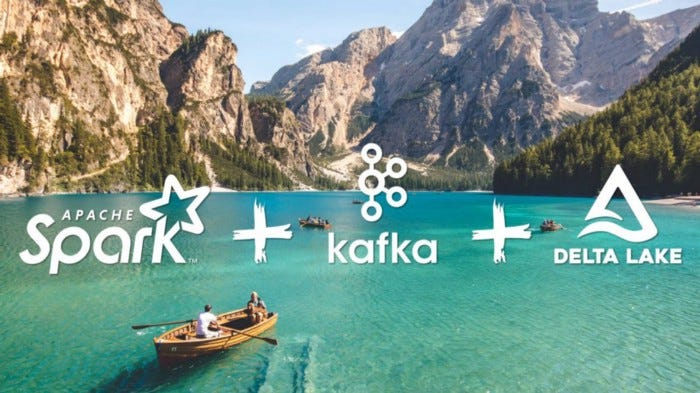# Using Spark Streaming to merge/upsert data into a Delta Lake with working code

October 12, 2022

## Using Spark Streaming to merge/upsert data into a Delta Lake with working codeThis blog will discuss how to read from a Spark Streaming and merge/upsert data into a Delta Lake. We will also optimize/cluster data of the delta table. In the end, we will show how to start a streaming pipeline with the previous target table as the source.

Overall, the process works in the following manner, we read data from a streaming source and use this special function ***foreachBatch. ***Using this we will call any user-defined function responsible for all the processing. This function encapsulates the Merge and *Optimize *to the target Delta table.

First, we need some input data to merge. You could technically make a stream out of Kafka, Kinesis, s3, etc. for simplicity. Let’s generate a stream using the below. Feel free to alter numPartitions & rowsPerSecond . These parameters help you control how much volume of data you want to generate. In the below code, we generated 10,000 rows per second across 100 partitions.

## Generate streaming data at your desired rate

``````generated_df = (
.format("rate")
.option("numPartitions", 100)
.option("rowsPerSecond", 10 * 1000)
.selectExpr(
"md5( CAST (value AS STRING) ) as md5"
,"value"
,"value%1000000 as hash"
)
)

#display(generated_df)
``````

## Parameters / Variables (Feel free to change as per your needs)

``````target_table_name = "to_be_merged_into_table"
check_point_location = f"/tmp/delta/{target_table_name}/_checkpoints/"
join_column_name ="hash"
``````

## Create an Empty Delta table so data could be merged into it

``````spark.sql(f"""  DROP TABLE IF EXISTS {target_table_name};""")
(
generated_df.writeStream
.format("delta")
.outputMode("append").trigger(once=True)
.option("checkpointLocation", check_point_location)
.toTable(target_table_name)
)
``````

Check if data is populated

``````display(spark.read.table(target_table_name))
``````

## A user-defined function which does the data processing, Merge & Optimize

``````def make_changes_using_the_micro_batch(microBatchOutputDF, batchId: int):
print(f"Processing batchId: {batchId}")
spark_session_for_this_micro_batch = microBatchOutputDF._jdf.sparkSession()
spark_session_for_this_micro_batch.sql(f"""
SELECT *
FROM (
select *
,rank() over(partition by {join_column_name} order by value desc) as dedupe
)
WHERE
dedupe =1
spark_session_for_this_micro_batch.sql(f"""
MERGE INTO {target_table_name} target
on u.{join_column_name} = target.{join_column_name}
WHEN MATCHED THEN UPDATE SET *
WHEN NOT MATCHED THEN INSERT *
""")
optimize_every_n_batches = 20
#Define how often should optimize run? for example: at 50, it means that we will run the optimize command every 50 batches of stream data
if batchId % optimize_every_n_batches == 0:
optimize_and_zorder_table(table_name = target_table_name,  zorder_by_col_name = join_column_name)
``````

## Optimize/ Z-order a Delta table

Why do we need to optimize a table? If we keep adding files to our Delta table and never optimize/sort them then over time we need to read a lot of files during merge time. Thus, optimizing the Delta table after every N merges is better. N needs to be decided on your latency requirements. You could start with N as 10 and change it as per your needs.

The below code will run an optimize and zorder command on a given table that is being fed by a stream. Optimize commands can’t run in a silo because it will require us to pause and then resume the stream. Therefore, we need to call this function a part of the upsert function. This enables us to optimize before the next batch of streaming data comes through.

``````from timeit import default_timer as timer

def optimize_and_zorder_table(table_name: str, zorder_by_col_name: str) -> None:
"""
Parameters:
table_name: str
name of the table to be optimized
zorder_by_col_name: str
comma separated list of columns to zorder by. example "col_a, col_b, col_c"
"""
start = timer()
print(f"Met condition to optimize table {table_name}")
sql_query_optimize = f"OPTIMIZE  {table_name} ZORDER BY ({zorder_by_col_name})"
spark.sql(sql_query_optimize)
end = timer()
time_elapsed_seconds = end - start
print(
f"Successfully optimized table {table_name} . Total time elapsed: {time_elapsed_seconds} seconds"
)
``````

## Orchestrate from readStream -> Merge -> Optimize

``````(
generated_df
.writeStream.format('delta')
.trigger(processingTime='30 seconds')
.option("checkpointLocation", check_point_location)
.foreachBatch(make_changes_using_the_micro_batch)
.start()
)
``````

If you have reached so far, you should have an end-to-end pipeline working with streaming data and merging data into a Delta table.

As the next step, let’s use the previous target table as our new streaming source.

## Use the target table as a source for the next streaming pipeline

Change data feed allows Databricks to track row-level changes between versions of a Delta table. When enabled on a Delta table, the runtime records change events for all the data written into the table. This includes the row data along with metadata indicating whether the specified row was inserted, deleted, or updated.

``````spark.sql(f'''
ALTER TABLE {target_table_name} SET TBLPROPERTIES (delta.enableChangeDataFeed=true)
''')
``````

## Reading change data as a stream

``````display(